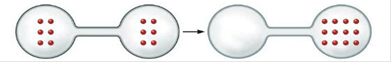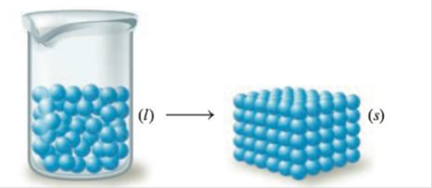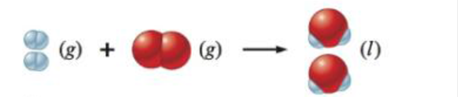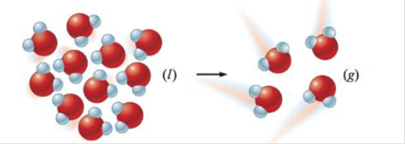Chapter 17, Problem 41E

Chapter
Section
Textbook Problem

# Predict the sign of ∆S° for each of the following changes. Assume all equations are balanced.a.b.c.d.

(a)

Interpretation Introduction

Interpretation: The sign of ΔSo is to be predicted in each case.

Concept introduction: Entropy is the measure of degree of disorder or randomness. Increase in the randomness of the system leads to increase in the entropy (entropy is positive).

Explanation

Explanation

The given process is,

Figure 1

The molecules on reactant side are more randomly arranged as compared to product side...

(b)

Interpretation Introduction

Interpretation: The sign of ΔSo is to be predicted in each case.

Concept introduction: Entropy is the measure of degree of disorder or randomness. Increase in the randomness of the system leads to increase in the entropy (entropy is positive).

(c)

Interpretation Introduction

Interpretation: The sign of ΔSo is to be predicted in each case.

Concept introduction: Entropy is the measure of degree of disorder or randomness. Increase in the randomness of the system leads to increase in the entropy (entropy is positive).

(c)

Interpretation Introduction

Interpretation: The sign of ΔSo is to be predicted in each case.

Concept introduction: Entropy is the measure of degree of disorder or randomness. Increase in the randomness of the system leads to increase in the entropy (entropy is positive).

### Still sussing out bartleby?

Check out a sample textbook solution.

See a sample solution

#### The Solution to Your Study Problems

Bartleby provides explanations to thousands of textbook problems written by our experts, many with advanced degrees!

Get Started